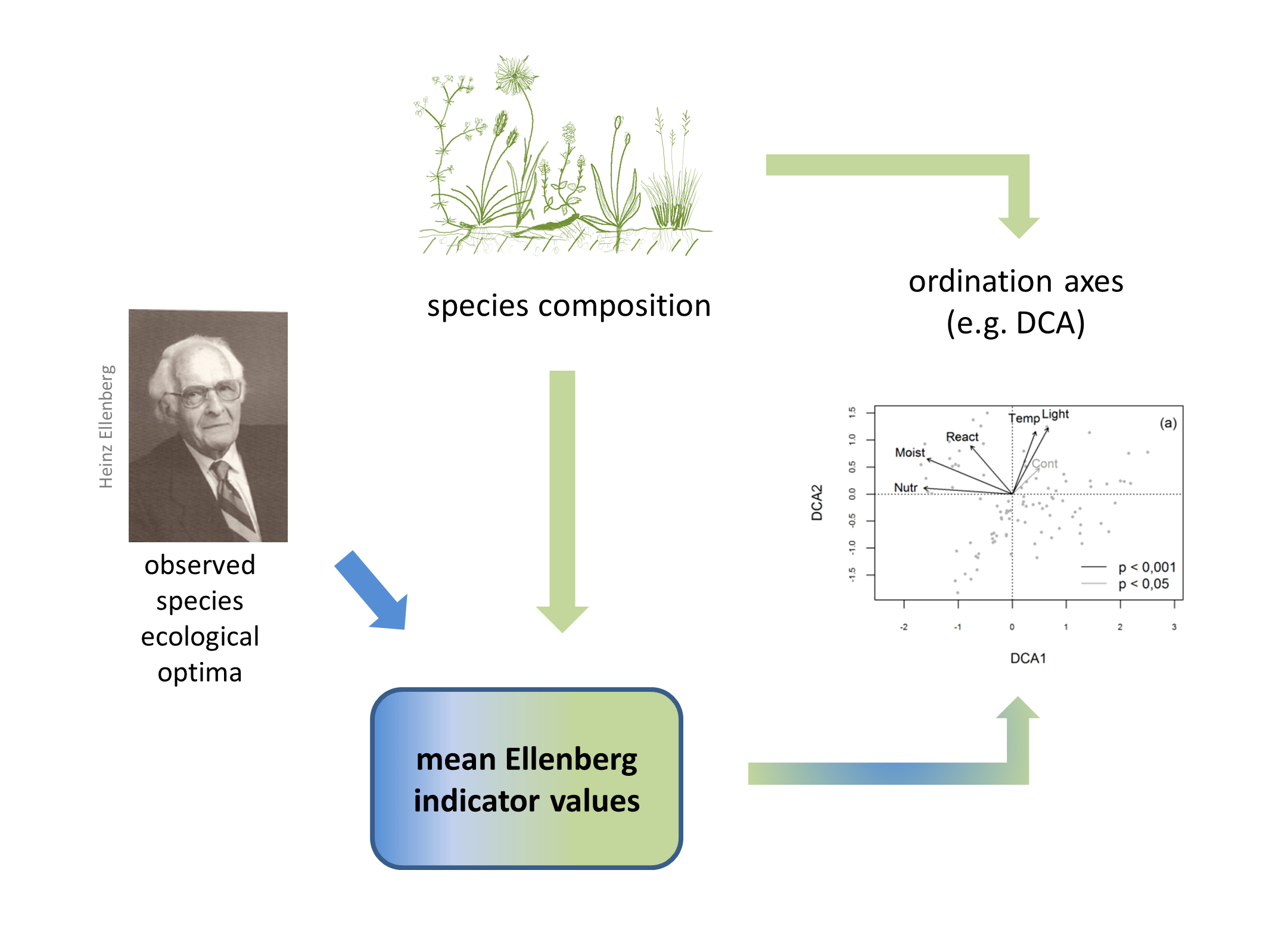I started to think more intensively about Ellenberg indicator values issue at one conference while listening to the presentation, where a colleague used mean Ellenberg indicator values as explanatory variables in constrained ordination. I considered this as a kind of statistical heresy, perfect example of circularity of reasoning – you take your vegetation data, calculate mean Ellenberg indicator values for each plot, and in turn use these mean values to explain the original data. But it’s tempting – mean Ellenberg values are often considered as good proxies for measured environmental variables, and they are easy to calculate, so using them as explanatory variables is attractive. I tried that – I took a dataset with measured soil pH and calculated mean Ellenberg values for soil reaction, and compared how much variation in species data will be explained by pH and how much by mean Ellenberg; Ellenberg was a way better predictor than measured pH. Ok, so here we have the consequence of circularity. Thinking it through, I concluded that the reason is that mean Ellenberg values carry legacy of the species composition, from which they were calculated – if two plots have the same species composition, their mean Ellenberg values will be identical (considering mean not weighted by species covers), and if the species composition differ a bit, Ellenberg will change just slightly (changing one or a few numbers while calculating the mean doesn’t change the result too much).